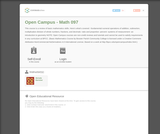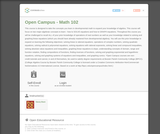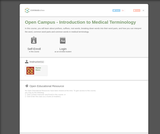# 5 Results

View
Selected filters:
• Bossier Parish Community CollegeOnly Sharing Permitted
CC BY-NC-ND
Rating

This course is a review of basic mathematics skills. Here's what's covered:
-fundamental numeral operations of addition, subtraction, multiplication
division of whole numbers, fractions, and decimals
-ratio and proportion
-percent
-systems of measurement
-an introduction to geometry
NOTE: Open Campus courses are non-credit reviews and tutorials and cannot be used to satisfy requirements in any curriculum at BPCC. (Basic Mathematics Course by Bossier Parish Community College is licensed under a Creative Commons Attribution-NonCommercial-NoDerivatives 4.0 International License.
Based on a work at http://bpcc.edu/opencampus/index.html.)

Subject:
Mathematics
Material Type:
Full Course
Provider:
Bossier Parish Community College
Author:
Michelle Barnickel
10/11/2017Only Sharing Permitted
CC BY-NC-ND
Rating

In this beginning algebra course, you'll learn about
fundamental operations on real numbers
exponents
solving linear equations and inequalities
applications
functions
graphing linear equations
slope
systems of linear equations

Subject:
Algebra
Material Type:
Full Course
Lecture
Lesson
Module
Provider:
Bossier Parish Community College
Author:
Gail Hendrix
10/16/2017Only Sharing Permitted
CC BY-NC-ND
Rating

This course is designed to take the concepts you learn in developmental math to expand your knowledge of algebra. This course will focus on two major algebraic concepts to learn - how to SOLVE equations and how to GRAPH equations. Throughout this course you will be challenged to recall ALL of your prior knowledge of operations of real numbers as well as your knowledge related to solving and graphing linear equations (which you should have already mastered from developmental algebra). You will use this prior knowledge to expand on learning the following objectives: solving linear & rational equations. operations of complex numbers, solving quadratic equations, solving radical & polynomial equations, solving equations with rational exponents, solving linear and compound inequalities, solving absolute value equations and inequalities, graphing linear equations & slope, understanding concepts of domain, range and function notation, finding compositions of functions, finding inverses of functions, solving and graphing exponential and logarithmic equations, solving and graphing systems of equations and inequalities, and graphing conics.

*Open Campus courses are non-credit tutorials and cannot, in and of themselves, be used to satisfy degree requirements at Bossier Parish Community College (BPCC). (College Algebra Course by Bossier Parish Community College is licensed under a Creative Commons Attribution-NonCommercial-NoDerivatives 4.0 International License. Based on a work at http://bpcc.edu/opencampus/index.html.)

Subject:
Mathematics
Algebra
Material Type:
Full Course
Provider:
Bossier Parish Community College
Author:
Stacey Black
10/11/2017Only Sharing Permitted
CC BY-NC-ND
Rating

In this course, you will learn about prefixes, suffixes, root words, breaking down words into their word parts, and how you can interpret the word, common word parts and common words in medical terminology.

Subject:
Health, Medicine and Nursing
Material Type:
Full Course
Provider:
Bossier Parish Community College
Author:
Rachel Basco
10/16/2017Only Sharing Permitted
CC BY-NC-ND
Rating

These Trigonometry lecture videos coterminal angles, trig functions, quadrantal angles, special acute angles, co-functions, finding theta, reference angles, trig functions, radian measure, arc length, area of a sector, graphing sine and cosine using t-table, amplitude and frequency, phase shift for sine and consine, vertical shift, tangent curve, cotangent transformations, evaluating trig identities, trig expressions, sum and difference for cosine, double and half angle identities, inverse, principal values, solving difficult trig equations, law of cosines, area of a triangle, and vectors and bearing.

Subject:
Trigonometry
Material Type:
Lecture
Provider:
Bossier Parish Community College
Author:
Stacey Black